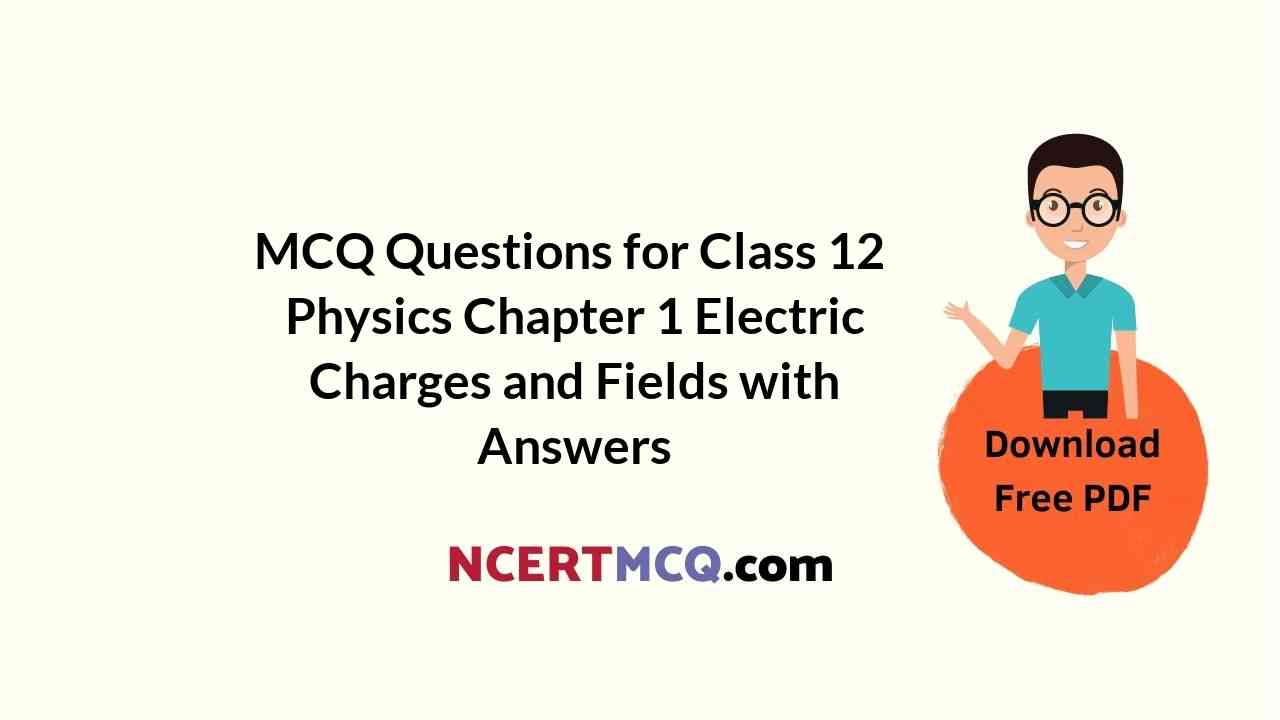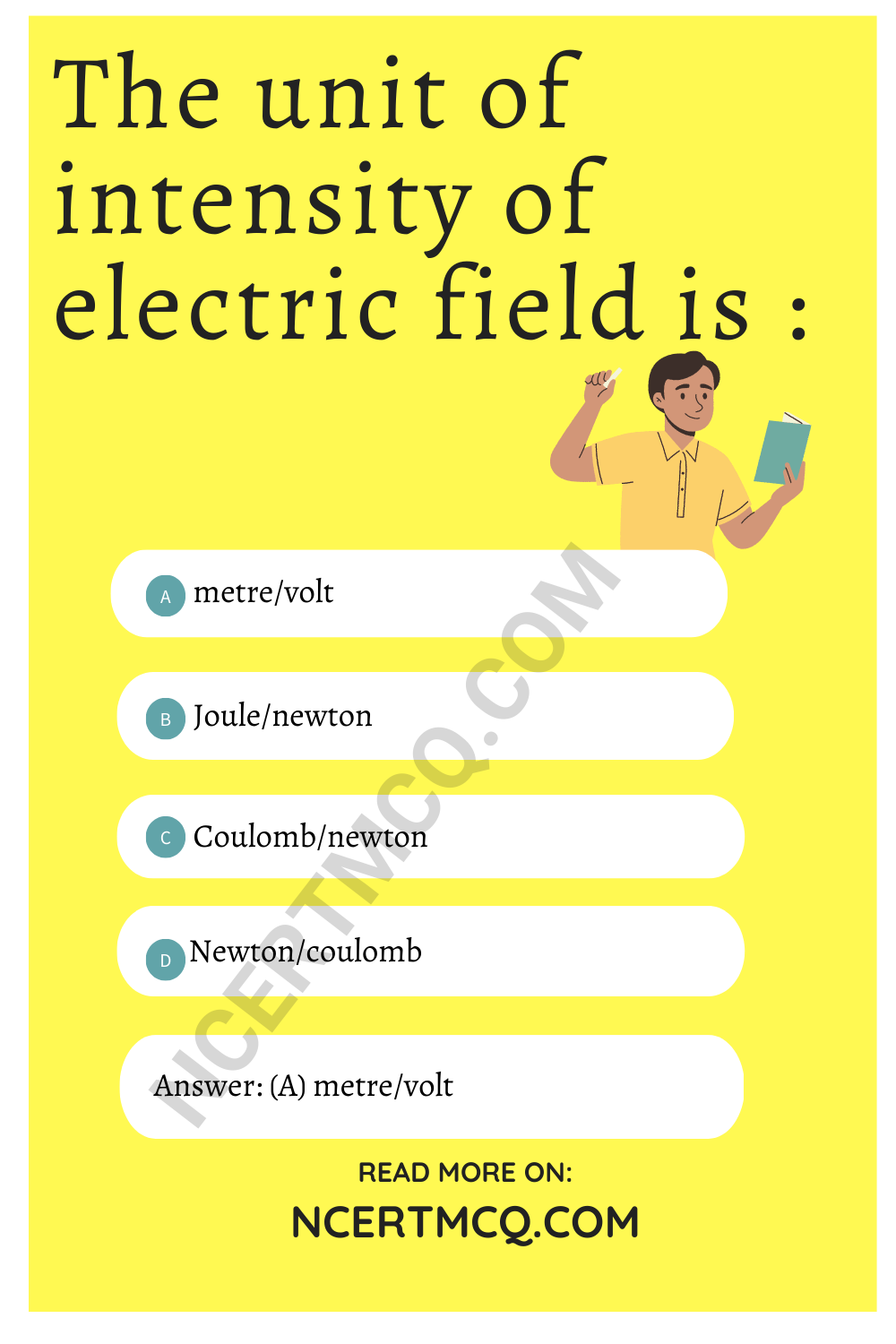Check the below NCERT MCQ Questions for Class 12 Physics Chapter 1 Electric Charges and Fields with Answers Pdf free download. MCQ Questions for Class 12 Physics with Answers were prepared based on the latest exam pattern. We have provided Electric Charges and Fields Class 12 Physics MCQs Questions with Answers to help students understand the concept very well.

## Electric Charges and Fields Class 12 MCQs Questions with Answers

Class 12 Physics Chapter 1 MCQ Question 1.
Which one of the following is the unit of electric field?
(a) Coulomb
(b) Newton
(c) Volt
(d) N/C

Chapter 1 Physics Class 12 MCQ Question 2.
Three charges + 3q + q and Q are placed on a st. line with equal separation. In order to maket the net force on q to be zero, the value of Q will be :
(a) +3q
(b) +2q
(c) -3q
(d) -4q

Physics Class 12 Chapter 1 MCQ Question 3.
If an electric dipole is kept in a uniform electric field then resultant electric force on it is :
(a) always zero
(b) never zero
(c) depend upon capacity of dipole
(d) None

Electric Charges And Fields Class 12 MCQ Question 4.
The number of electron-taken out from a body to produce 1 coulomb of charge will be :
(a) 6.25 × 1018
(b) 625 × 1018
(c) 6.023 × 1023
(d) None

Class 12 Physics Chapter 1 MCQ Questions And Answers Question 5.
The work done in rotating an electric dipole in an electric field:
(a) W = ME (1 – cos θ)
(b) W = ME tan θ
(c)W = ME sec θ
(d) None

Answer: (a) W = ME (1 – cos θ)

Physics Chapter 1 Class 12 MCQ Question 6.
If sphere of bad conductor is given charge then it is distributed on:
(a) surface
(b) inside the surface
(c) only inside the surface
(d) None

Ch 1 Physics Class 12 MCQ Question 7.
Electric field in a cavity of metal:
(a) depends upon the surroundings
(b) depends upon the size of cavity
(c) is always zero
(d) is not necessarily zero

Answer: (d) is not necessarily zero

MCQ Of Chapter 1 Physics Class 12 Question 8.
The dielectric constant of a metal is:
(a) 0
(b) 1
(c) ∞
(d) -1

MCQ Physics Class 12 Chapter 1 Question 9.
1 coulomb is equal to:
(a) 3 × 109 e.s.u.
(b) $$\frac{1}{3}$$ × 109 e.s.u.
(c) 3 × 1010 e.s.u.
(d) $$\frac{1}{3}$$ × 1010 e.s.u.

Answer: (a) 3 × 109 e.s.u.

MCQ Questions Class 12 Physics Chapter 1 Question 10.
Each of the two point charges are doubled and their distance is halved. Force of interaction becomes p times, w here p is :
(a) 1
(b) 4
(c) $$\frac{1}{16}$$
(d) 16

Physics Chapter 1 MCQ Class 12 Question 11.
Coulomb’s law in vector form can be written as
$$\vec{F}$$ = ($$\frac{1}{4πε_n}$$) ($$\frac{q_1q_2}{r^2}$$)$$\vec{r}$$
where ε0 is the permitivity of free space. The SI units of ε0 will be:
(a) N-1m-2C-2
(b) Nm-2C2
(c) N-1m-2C9.
(d) Nm-2C2

MCQ On Electric Charges And Fields Pdf Question 12.
The dimensional representation of cu will be :
(a) [MLT4 A2]
(b) [M-1L-3T4A2]
(c)[ML-2T ]
(d) None of these

Electric Charges And Fields MCQ Question 13.
When placed in a uniform field, a dipole experiences:
(a) a net force
(b) a torque
(c) both a net force and torque
(d) neither a net force nor a torquePhysics Class 12 MCQ Chapter 1 Question 14.
A parrot comes and sits on a bare high power line. It will:
(a) experience a mild shock ‘
(b) experience a strong shock.
(c) get killed instantaneously
(d) not be affected practically

Answer: (d) not be affected practically

MCQ Of Physics Class 12 Chapter 1 Question 15.
The SI units of electric dipole moment are:
(a) C
(b) Cm-1
(c) Cm
(d) Nm-1

Class 12 Physics Ch 1 MCQ Question 16.
If two conducting sphere are connected after charging separately then:
(a) Electrostatic energy sphere energy will remain conserved
(b) Electrostatic energy charges remains conserved
(c) Electrostatic energy energy and charge remains conserved
(d) None

Answer: (b) Electrostatic energy charges remains conserved

MCQ Chapter 1 Physics Class 12 Question 17.
A soap bubble is given a negative charge, then its radius:
(a) increases
(b) decreases
(c) remains unchanged
(d) may increase or decrease

Class 12th Physics Chapter 1 MCQ Question 18.
In non-uniform electric field, electric dipole experiences:
(a) torque only
(b) torque as well as net force
(c) force only
(d) None of these

Answer: (b) torque as well as net force

Chapter 1 MCQ Physics Class 12 Question 19.
An electron is sent in an electric field of magnitude 9.1 × 106NC-1. The acceleration produced in it is :
(a) 1.6 ms-2
(b) 1.6 × 1018ms-2
(c) 3.2 × 1018ms-2
(d) 0.8 × 1018 ms-2

Electric Charge And Field MCQ Question 20.
A semicircular arc of radius r is charged uniformly and the charge per unit length is λ. The electric field at the centre is:
(a) $$\frac{λ}{2λε_0r^2}$$
(b) $$\frac{λ}{4πε_0r}$$
(c) $$\frac{λ^2}{2πε_0r}$$
(d) $$\frac{λ}{2πε_0r}$$

Answer: (b) $$\frac{λ}{4πε_0r}$$

Question 21.
When a dipole of moment $$\vec{p}$$ is placed in uniform electric field $$\vec{E}$$, then the torque acting on the dipole is:
(a) $$\vec{τ}$$ = $$\vec{p}$$.$$\vec{E}$$
(b) $$\vec{τ}$$ = $$\vec{P}$$ + $$\vec{E}$$
(c) $$\vec{τ}$$ = $$\vec{p}$$ + $$\vec{E}$$
(d ) $$\vec{τ}$$ = $$\vec{p}$$ – $$\vec{E}$$

Answer: (c) $$\vec{τ}$$ = $$\vec{p}$$ + $$\vec{E}$$

Question 22.
Two point charges +8q and -2q are located at ,v = O and ,v = L respectively. The location of a point on the .v-axis at which the net electric field due to these two point charges b is zero :
(a) 4L
(b) 8L
(c) L/4
(d) 2L

Question 23.
The graph drawn between V are r for a non-conducting charged solid sphere of radius R for r < R will be :
(a) straight line
(b) parabola
(c) hyperbola
(d) None of these

Question 24.
Corona discharge takes place:
(a) at the surface of a conductor
(b) near the sharp points of a conductor
(c) outside the conductor
(d) at the centre of the conductor

Answer: (b) near the sharp points of a conductor

Question 25.
The belt of a Vande Graaf generator gets charge by :
(a) corona discharge
(b) induction
(c) electrification
(d) None of these

Question 26.
Quantisation of charge implies:
(a) Charge does not exist
(b) Charge exists on particles
(c) There is a minimum permissible magnitude of charge
(d) Charge can’t be created

Answer: (c) There is a minimum permissible magnitude of charge

Question 27.
The conservation of electric charge implies that:
(a) Charge can’t be created
(b) Charge can’t be destroyed
(c) The number of charged particle in the universe is constant
(d) Simultaneous creation of equal and opposite charges is permissible

Answer: (d) Simultaneous creation of equal and opposite charges is permissible

Question 28.
The number of electrons contained in one coulomb of charge is:
(a) 6.25 × 1018
(b) 6.25 × 1017
(c) 6.25 × 1019
(d) 1.6 × 1019

Question 29.
The unit of intensity of electric field is :
(a) metre/volt
(b) Joule/newton
(c) Coulomb/newton
(d) Newton/coulombQuestion 30.
1 V equals to:
(a) 1 J
(b) 1 JC-1
(c) 1 CJ-1
(d) 1 JC

Question 31.
1 V m-1 equals to:
(a) 1 N
(b) 1 Mm-1
(c) 1 NC-1
(d) 1 J-1

Question 32.
The minimum amount of charge observed so far is:
(a) 1 C
(b) 4.8 × 1-13 C
(c) 1.6 × 10-19 C
(d) 1.6 × 1019 C

Answer: (c) 1.6 × 10-19 C

Question 33.
The ratio of electric force between two electrons to the gravitational force between them is of the order:
(a) 1042
(b) 1039
(c) 1036
(d) 1